# Formula Columns

Together with table columns containing simple values (for example, of the numeric or text type), TeamDesk allows a user to create columns with formula values. The value for such columns is set as a result of configuration or calculation by predefined formulas.

Formula-defined columns are created in the same way as columns of ordinary types (see the Creating new columns section). For columns that contain formulas, you may use the following data types:

 Column Type Formula Description Formula – Text This type of columns allows formulas that provide a text result. The formula may contain a text literal, variable or references to columns that store text values. For example, in the “Name” formula-text column you can combine a first name value and a last name value kept in corresponding columns. In this case the List function is helpful: List(“ ”, [First Name], [Last Name]). Formula – Number This type allows formulas that provide a numeric result: integer, decimal, positive or negative. The formula may contain actual numbers (literals), references to columns that store numeric values or variables that should be converted first using the ToNumber() function. If you need to calculate the Age using the Birthdate value, insert the following formula into the formula-numeric column: Year(Today()) - Year([Birthdate]) - If(DayOfYear(Today()) < DayOfYear([Birthdate]), 1, 0) Formula – Date This type allows formulas that provide a date. If in the formula you use a literal or variable, convert it into a date first using the ToDate() function. To retrieve today’s date, use the Today() function. Formula – Time This type allows formulas that are used to display time of a day. The result may be created by retrieving time from a Timestamp column or by constructing time with the help of literal values or column references. Formula – Duration This type allows formulas that are used to calculate a certain period of time. To calculate a duration formula, you may choose from the list of Duration functions. In addition, a duration formula may be composed by subtracting one value from another: Timestamp from Timestamp Date from Date TimeofDay from TimeofDay The calculation result may be positive or negative. Formula – Checkbox Checkbox formula always provides the ‘Yes’ (check box is selected if the expression is true for the record) or ‘No’ response (check box is not selected if the expression is false). For example, when tracking project deadlines, the [Project End Date] < Today() formula in the Deadline Expired column, shows whether the team fails to meet a deadline (Yes), or there is still time left for development (No). Formula – Phone Number This type allows formulas that are used to make up a phone number out of the area code, phone number and maybe extension. Use text values for formula arguments: literals, variables or column references. For example, in the List(“-”, [Area Code], [Phone Number], [Ext.]) formula, area code, phone number and extension number values are retrieved from corresponding columns. TeamDesk parses data from Phone and Formula-Phone columns. The system decorates them as Skype links on desktops and tablets and as links to invoke phone dialer on mobile phones. Skype requires phone numbers to contain country code. If the column data is missing country code, TeamDesk infers the code from database Language and Locale settings. When a text is entered and stored in a Phone Number column, it is decorated as a link. Also, there is the tooltip that displays the phone number formatted according to your country rules. Formula – E-mail Address This type allows formulas used to create an e-mail address either by entering a textual literal in double quotes directly (for example, “info@mywebsite.com”) or by drawing up various column values together (for example, List(“@“, [Local E-mail Part], [domain])). Use text values for formula arguments. In the user mode, the calculated formula result will be represented as a mailto hyperlink used to create a new e-mail message that will be sent to the specified recipient. Formula – URL This type of formulas, like formulas for the E-mail Address field, can be made up either by entering a textual literal directly (“www.teamdesk.net”) or by composing the URL from constituent parts, like variables, literals, column references and other text values. Additionally, the following functions may be used to construct a URL formula: URLRoot(), BackURL(), AppId(), TableId(), RecordId(). Formula – User This type of columns allows to provide names of registered database users. The formula may contain column references or text literals converted into the required data format. Formula-Barcode Any text value can be displayed as a barcode. The formula-barcode column allows to generate barcodes that can be inserted into the document and scanned. Formula-Location This type of column options match the Location column. Also, there are couple of functions you use with locations: IsNull(location) checks whether location field is blank. Nz(location, location, …) – to choose first non-blank location from the list ToLocation(latitude, longitude) – constructs the location from a pair of coordinates ToLocation(text) – constructs the location from text (comma separated pair of decimal numbers) Latitude(location) – extracts latitude Longitude(location) – extracts longitude Distance(location, location, “unit”) – calculates distance between two locations on elliptic Earth. Result is returned in meters, by default or according to the unit provided: “m” for meters, “km” for kilometers or “mi” for miles. Finally, there is a DeviceLocation() function for use in place of pair DeviceLatutude() and DeviceLongitude() in actions designed for mobile apps.

After a column of a required type has been created, it is necessary to specify the formula itself in the properties of a created column.

To set a new formula:

Click the Setup link at the top right corner of the window.

Select a table you need.

From the setup menu select Columns > Customize existing columns. The system will display the Columns form;

Click the Edit button next to a newly created formula defined column;

In the Formula field set a new formula according to which the value of a column will be set.Click ‘[’ to list all columns of a table and insert column variables you need. Also you can type first letters of the column name after ‘[’ sign and the columns starting with these letters will be displayed in the dropdown.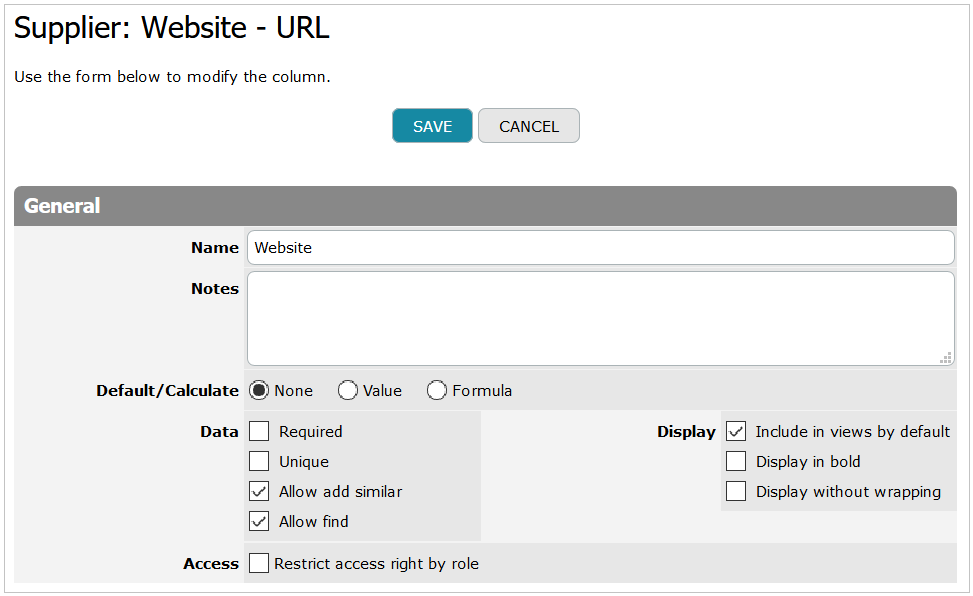Click Ctrl+Space to list function names and select functions you need.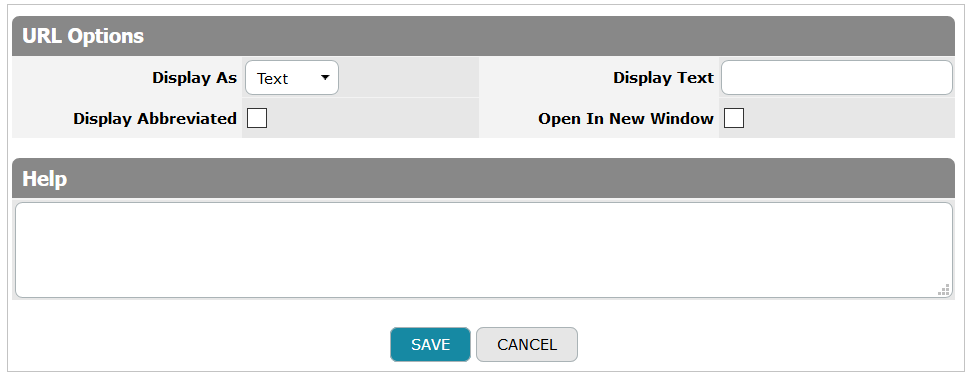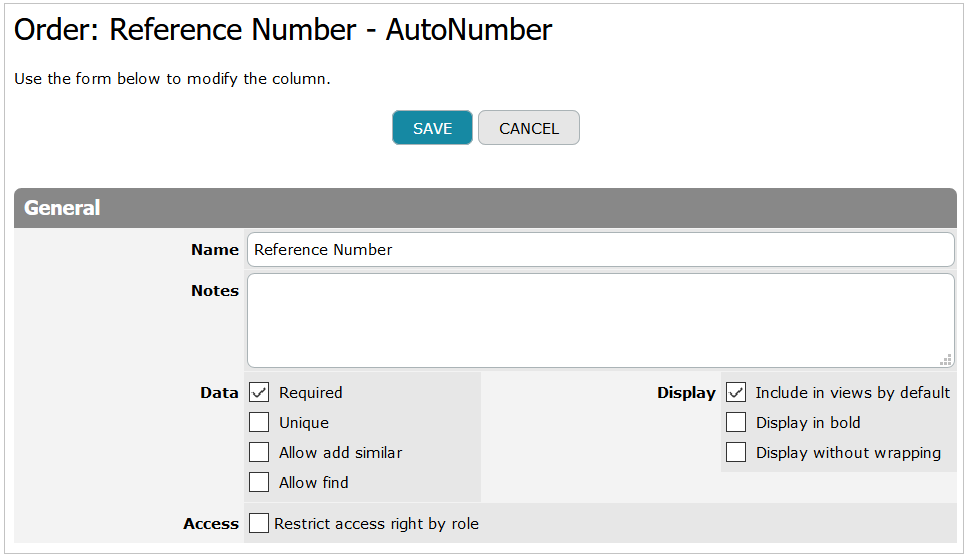To view a full list of functions used for formula generating, see Formula Language Reference.

Wherever you need information from User Properties related to a current user, you can simply refer to a column of the User Property table by its name. Just click ‘[’ to list all columns of a table and choose a column from the User Properties section. Also, you can type first letters of the column name after ‘[’ sign and the columns starting with these letters will be displayed in the dropdown.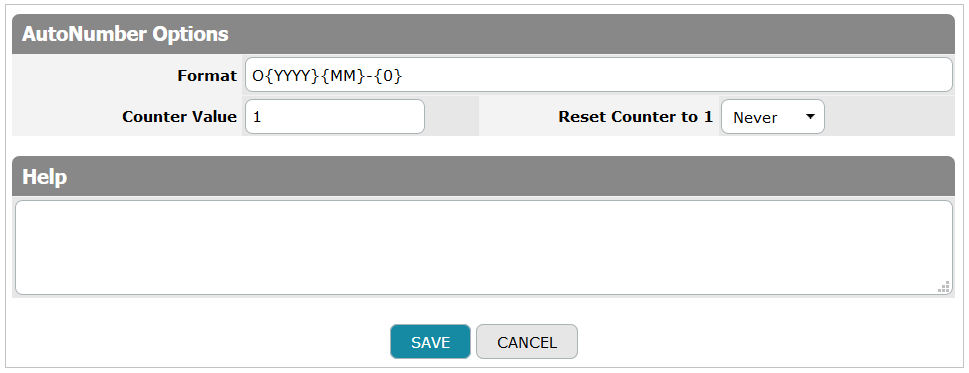You can colorize values stored in the Formula-Text columns. Just use the Colorization checkbox displayed in the Advanced Options. Colorization bases on whether the value contains certain text. More details are described in the Column Value Colorization section.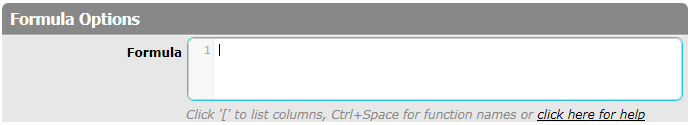The last option is the Help field.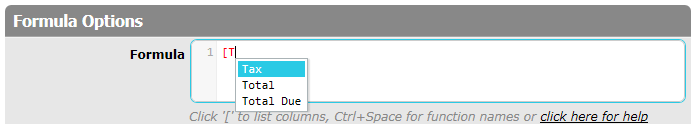When this Help text property is filled in, the question mark is displayed near the field name on the View/Edit form. If you point to this question mark, the pop-up with a tip or a help text message will be shown to a user.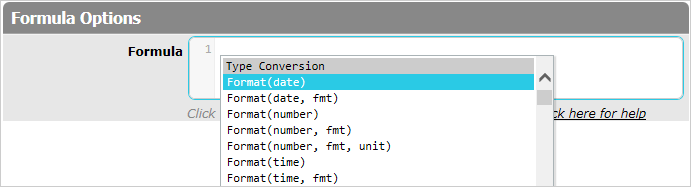Next: Formula-XHTML